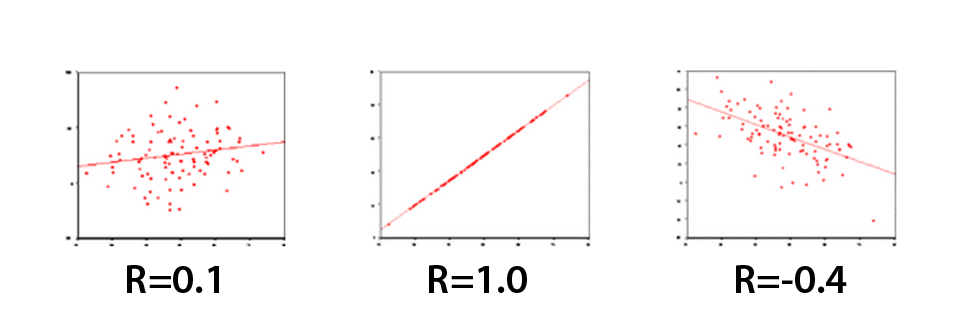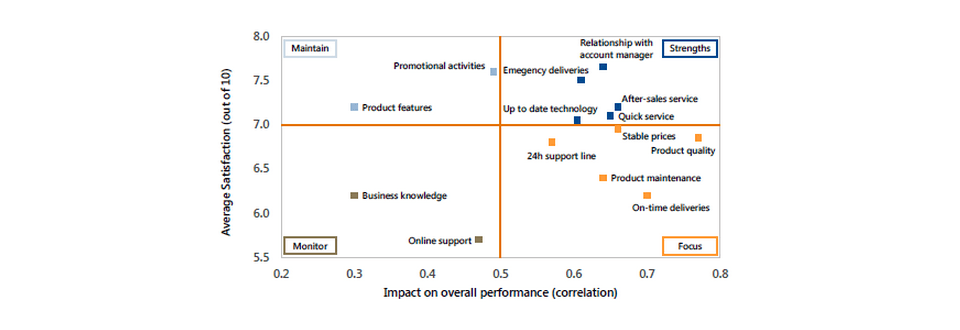Find out what satisfies your customers and employees – and what keeps them loyal. Typically used in customer satisfaction and employee satisfaction studies, correlation analysis measures the degree of linear relationship between two variables.

## B2b correlation analysis

It answers questions like: “which elements contribute most to customer/employee overall satisfaction or loyalty?” This leads to a ‘derived importance versus satisfaction’ map – see below. Correlation analysis is ideal when sample sizes are too low (e.g. less than 100) to run a regression analysis.

In regression analysis, the emphasis is on predicting one variable from the other whereas in correlation analysis, the emphasis is the degree to which a linear model may describe the relationship between two variables.

The correlation coefficient may take on any value between + and – 1. The sign of the correlation coefficient (+, -) defines the direction of the relationship, either positive or negative. A positive correlation coefficient means that, as the value of one variable increases, the value of the other variable increases; as one decreases the other decreases. A negative correlation coefficient indicates that as one variable increases, the other decreases, and vice-versa.

The absolute value of the correlation coefficient measures the strength of the relationship. A correlation coefficient of r=0.50 indicates a stronger degree of linear relationship than one of r=0.40. Thus a correlation coefficient of zero (r=0.0) indicates the absence of a linear relationship and correlation coefficients of r=+1.0 and r=-1.0 indicate a perfect linear relationship.

The scatter plots presented below perhaps best illustrate how the correlation coefficient changes as the linear relationship between the two variables is altered. When r=0.0 the points scatter widely about the plot, the majority fall roughly in the shape of a circle. As the linear relationship increases, the circle becomes more and more elliptical in shape until the limiting case is reached (r=1.00 or r=-1.00) and all the points fall on a straight line. A number of scatter plots and their associated correlation coefficients are presented below: